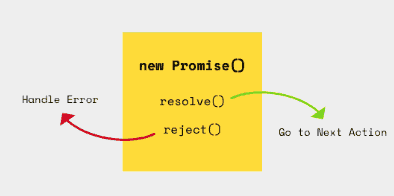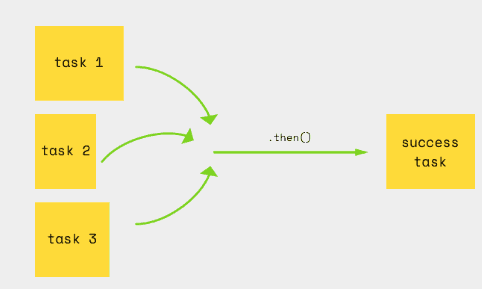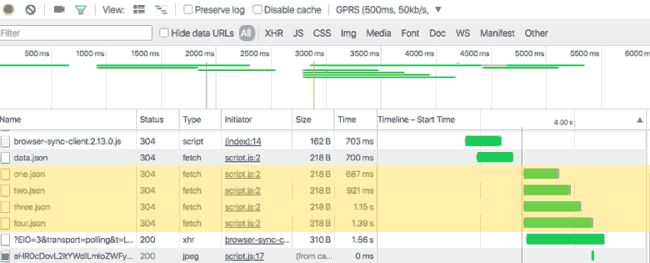# Javascript/ES6 Promise用法

Javascript Promise代表一个操作的结果还没有完成，将在未来某个时间有确定结果，比如网络请求操作，当我们从某个数据源抓取数据时，实际上没有办法绝对地知道什么时候能够接受到响应。

```doSomething(function(`responseOne `)`  `{```
```doSomethingElse(`responseOne `,`  `function(`responseTwo `,` err `)`  `{```
```if`  `(`err `)`  `{`  `handleError(`err `);`  `}```
```doMoreStuff(`responseTwo `,`  `function(`responseThree `,` err `)`  `{```
```if`  `(`err `)`  `{`  `handleAnotherError(`err `);`  `}```
```doFinalThing(`responseThree `,`  `function(`err `)`  `{```
```if`  `(`err `)`  `{`  `handleAnotherError(`err `);`  `}```
```// Complete```
```});`  `// end doFinalThing```
```});`  `// end doMoreStuff```
```});`  `// end doSomethingElse```
```});`  `// end doSomething ```

Promise提供标准的干净的按照顺序发生的任务：

```doSomething()```
```.then(`doSomethingElse `)```
```.catch(`handleError `)```
```.then(`doMoreStuff `)```
```.then(`doFinalThing `)```
```.catch(`handleAnotherError `) ```

### 创建Promise

`var` promise  `=`  `new`  `Promise(`  `function(`resolve `,` reject `)`  `{`  `/* Promise content */`  `}`  `)`  ` ````function`  `get(`url `)`  `{```
```return`  `new`  `Promise(function(`resolve `,` reject `)`  `{```
```var` req  `=`  `new`  `XMLHttpRequest();```
req ```.open('GET',` url `);```
req ```.`onload  `=`  `function()`  `{```
```if`  `(`req `.`status  `==`  `200)`  `{```
```resolve(`req `.`response `);`  `/* PROMISE RESOLVED */```
```}`  `else`  `{```
```reject(Error(`req `.`statusText `));`  `/* PROMISE REJECTED */```
```}```
```};```

req ```.`onerror  `=`  `function()`  `{`  `reject(Error("Network Error"));`  `};```
req ```.send();```
```});```
```} ```

### 使用Promise

```get(`url `)```
```.then(function(`response `)`  `{```
```/* successFunction */```
```},`  `function(`err `)`  `{```
```/* errorFunction */```
```}) ```

### 处理错误

```get(`url `)```
```.then(function(`response `)`  `{```
```/* successFunction */```
```},` undefined `)```
```.then(`undefined `,`  `function(`err `)`  `{```
```/* errorFunction */```
```}) ```

```get(`url `)```
```.then(function(`response `)`  `{```
```/* successFunction */```
```})```
```.catch(function(`err `)`  `{```
```/* errorFunction */```
```}) ```

### 链式chaining

```get(`url `)```
```.then(function(`response `)`  `{```
response  ```=` JSON `.parse(`response `);```
```var` secondURL  `=` response `.`data `.```url
```return`  `get(` secondURL  `);`  `/* Return another Promise */```
```})```
```.then(function(`response `)`  `{```
response  ```=` JSON `.parse(`response `);```
```var` thirdURL  `=` response `.`data `.```url
```return`  `get(` thirdURL  `);`  `/* Return another Promise */```
```})```
```.catch(function(`err `)`  `{```
```handleError(`err `);```
```}); ```

### 并行执行Promise

Promise.all()方法返回一个成功完成每个promise数组，如果任何这个数组中任何一个promise被拒绝也就是失败，Promise.all()会也拒绝或失败。

```var` arrayOfURLs  `=`  `['one.json',`  `'two.json',`  `'three.json',`  `'four.json'];```
```var` arrayOfPromises  `=` arrayOfURLs `.map(get);```

Promise ```.all(`arrayOfPromises `)```
```.then(function(`arrayOfResults `)`  `{```
```/* Do something when all Promises are resolved */```
```})```
```.catch(function(`err `)`  `{```
```/* Handle error is any of Promises fails */```
```}) ```Javascript/ES6主题